# Invariant generation methods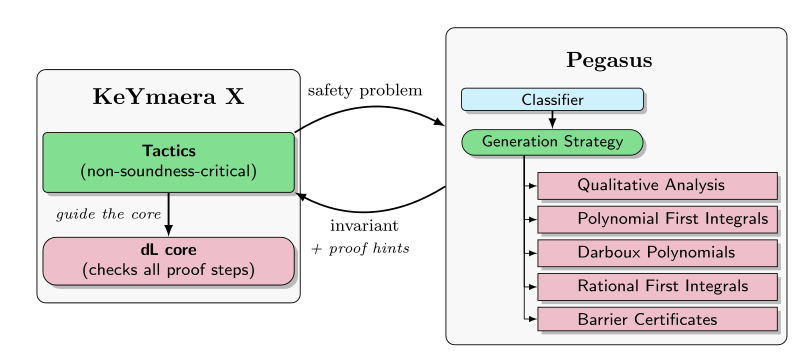As of version 1.1 Pegasus implements a number of methods for generating inductive invariants for continuous systems.
A more detailed description of these methods may be found in the FMSD article Pegasus: Sound Continuous Invariant Generation.

### Barrier certificates

The method of barrier certificates is a popular and promising technique for safety verification of continuous systems. It employs Lyapunov-like differential inequalities and typically requires a polynomial template which is then transformed into a constraint problem in the template coefficient variables. If the constraint is feasible, a real-valued polynomial $B\left(\mathbf{x}\right)$ can be synthesized from the feasible point, with the property that $B\left(\mathbf{x}\right)\le 0$ defines a continuous invariant of the system which separates the initial states of the system from states that are deemed unsafe. There are many different flavors of barrier certificates in the verification literature.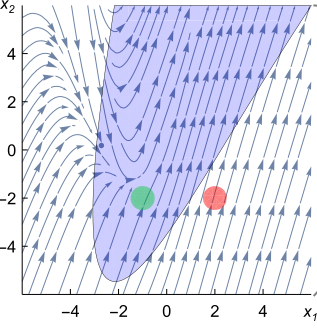A systematic development of the barrier certificate method and its integration with a sound theorem proving system is one of the goals behind developing Pegasus. As part of this effort, a generalization called vector barrier certificates was developed at the Logical Systems Lab in collaboration with Inria, which is based on the theory of comparison systems and serves to organize existing notions of barrier certificates under a unified framework.

### Polynomial First Integrals

First integrals, also known as conserved quantities, or constants of motion, are functions of state whose value remains constant as the system evolves. In other words, a first integral is a function $p$ whose time derivative is zero, i.e. $p\text{'}=0.$ The surfaces $p=0$ are invariant under the flow of an ODE, whose corresponding vector field is tangent to these surfaces.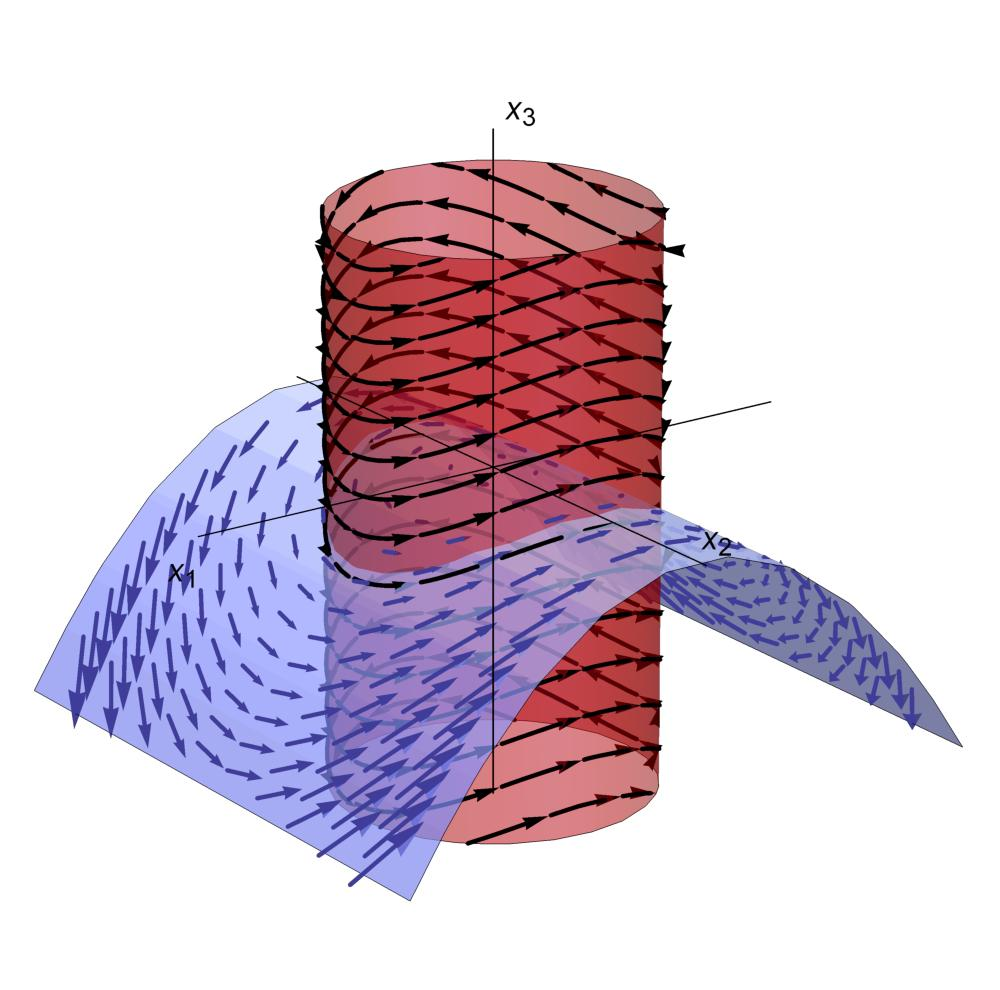Searching for polynomial first integrals can be achieved by using polynomial templates (up to some maximum polynomial degree) with symbolic monomial coefficients; these coefficients can be determined by solving a system of linear equations. However, polynomial first integrals are a very special case and in general first integrals can be very complicated functions that can be extremely difficult to find.

### Darboux polynomials and rational first integrals

Polynomials $p$ satisfying the differential equation $p\text{'}=\alpha p$ where $\alpha$ is also a polynomial are known as Darboux polynomials, named after Gaston Darboux who introduced them as a tool to study integrability. The roots of Darboux polynomials, i.e. states $\mathbf{x}$ satisfying $p\left(\mathbf{x}\right)=0$, represent an important class of algebraic invariants that can be used to decompose the state space of a system into disconnected invariant regions between which there is no flow. Besides their use in verification, Darboux polynomials are a crucial ingredient in the Prelle-Singer method for computing closed form elementary solutions to ODEs; as a consequence, algorithms for computing Darboux polynomials have been developed by researchers working in computer algebra long before the verification community began offering solutions to this problem. Pegasus implements algorithms for generating Darboux polynomials (up to a given bounded polynomial degree) and employs this capability to search for invariants in non-linear systems.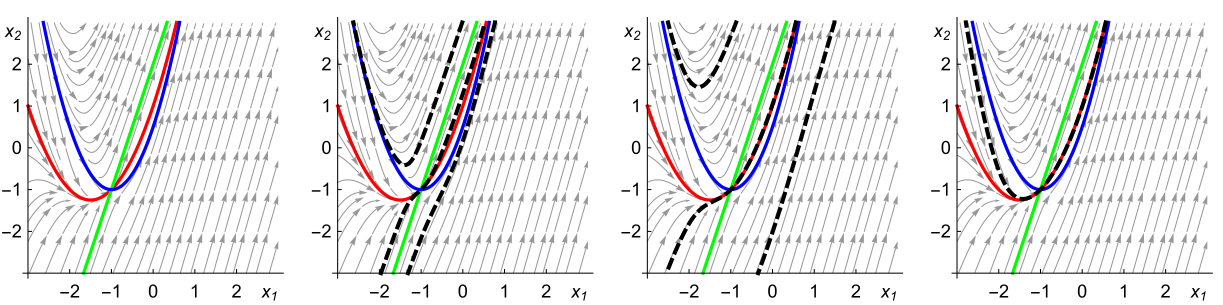Rational first integrals (i.e. first integrals that are rational functions) can sometimes be constructed from Darboux polynomials. Pegasus is able to search for rational first integrals of non-linear ODEs by searching for Darboux polynomials and attempting this construction.

Rational first integrals of linear systems with constant coefficients, i.e. systems of the form $\stackrel{\text{'}}{\mathbf{x}}=A\mathbf{x}$, where $A$ is an $n×n$ real matrix, can be computed using the so-called spectral method, which is based on the Darboux theory of integrability and makes use of the eigenstructure of the system matrix in order to construct first integrals. (The spectral method is described in the works of Falconi & Llibre, as well as Gorbuzov & Pranevich.) Linear systems with distinct real and rational eigenvalues present a particular interest, because they are algebraically integrable. Pegasus applies the spectral method to construct first integrals of linear systems.

### Qualitative abstraction

Qualitative abstraction of continuous systems is related to predicate abstraction. It produces a discrete abstraction by partitioning the state space of a continuous system using predicates that represent significant qualitative information about the system. If the set of predicates used for the abstraction is good, many interesting properties about the behavior of the continuous system may be proved.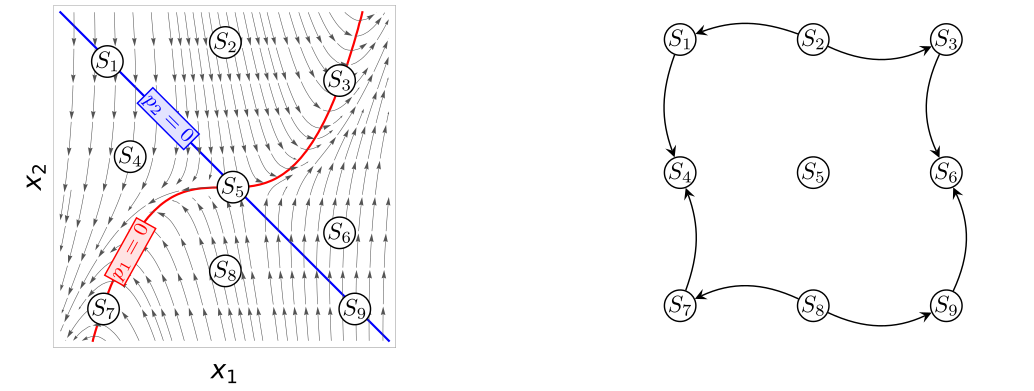The idea of qualitative abstraction originally emerged in the field of Artificial Intelligence in the 1980s and was used for qualitative simulation and qualitative reasoning about physical systems. Later work in this field in the 1990s considered verification of termporal properties of physical systems as an application of this technique. Within the verification community, qualitative abstraction was advanced in the work of A. Tiwari and was employed in tools such as HybridSAL from SRI International to enable model checking hybrid systems.

# Strategies for invariant generation

Pegasus implements strategies that generate invariants by combining invariant generation methods.

### Differential saturation

The main strategy for generating invariants for non-linear systems in Pegasus is based on a differential saturation loop, which works by refining the evolution constraint with invariants generated by a given sequence of generation methods.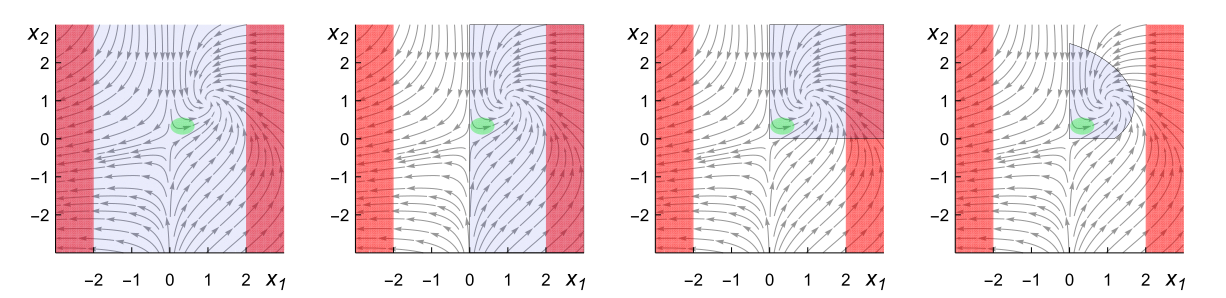Independent invariant generation sub-problems arise when algebraic invariants can be found. In these cases, the verification problem is decomposed into independent sub-problems that are solved separately.